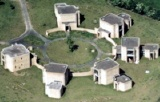# EPSRC Symposium Capstone Conference

### Speakers in order of talks

Massimo Fornasier (Massimo.Fornasier@oeaw.ac.at)

Mike Davies (Mike.Davies@ed.ac.uk) Sparse signal recovery: alternatives to L1

Finding sparse solutions to underdetermined inverse problems is a fundamental challenge encountered in a wide range of signal processing applications, from signal acquisition to source separation. Recent theoretical advances in our understanding of this problem have further increased interest in their application to various domains. In many areas, such as for example medical imaging or geophysical data acquisition, it is necessary to find sparse solutions to very large underdetermined inverse problems. Fast methods therefore have to be developed. In this talk, we will present fast algorithms that are competitive with the more classical convex L1 minimization (Basis Pursuit) technique. These methods will instead work more directly on the non-convex L0 optimisation function. One such strategy is the extremely simple algorithm called Iterative Hard thresholding (IHT). Despite its simplicity it can be shown that: it gives near-optimal performance guarantees; it is robust to observation noise; it has low bounded computational complexity per iteration and only requires a fixed number of iterations depending on the signal to noise ratio of the signal. Unfortunately a niaive application of IHT yields empirical performance substantially below that of other state of the art recovery algorithms. We therefore discuss have the algorithm can be modified to obtain performance that is comparable with state of the art while retaining its strong theoretical properties.

Rémi Gribonval (Remi.Gribonval@inria.fr) How many training samples does it take to learn a dictionary for sparse representations ?"

We consider the problem of learning a dictionary providing sparse representations for a given signal class, via $\ell_1$-minimisation. The problem can also be seen as factorising a $d \times N$ matrix $Y=(y_1 \ldots y_N), \, y_n\in \mathbb{R}^d$ of training signals into a $d \times K$ dictionary matrix $\mathbf{\Phi}$ and a $K \times N$ coefficient matrix $X=(x_1\ldots x_N),\, x_n \in \mathbb{R}^N$, which is sparse. The exact question studied here is when a dictionary coefficient pair $(\mathbf{\Phi},X)$ can be recovered as local minimum of a (nonconvex) $\ell_1$-criterion with input $Y=\mathbf{\Phi} X$. First, for general dictionaries and coefficient matrices, algebraic conditions ensuring local identifiability are derived, which are then specialised to the case when the dictionary is a basis. Finally, assuming a random Bernoulli-Gaussian sparse model on the coefficient matrix, it is shown that sufficiently incoherent bases are locally identifiable with high probability. The perhaps surprising result is that the typically sufficient number of training samples $N$ grows (up to a logarithmic factor) only linearly with the signal dimension, i.e. $N \approx C K \log K$, in contrast to previous approaches requiring combinatorially many samples.

Jared Tanner (jared.tanner@ed.ac.uk) Phase Transitions Phenomenon in Compressed Sensing

Compressed Sensing has broken the Nyquist barrier, but what is the sampling theorems for CS? Reconstruction algorithms typically exhibit a phase transition phenomenon for large problem sizes, where there is a domain of problem sizes for which successful recovery occurs with overwhelming probability, and there is a domain of problem sizes for which recovery failure occurs with overwhelming probability. These phase transitions serve as sampling theorems for CS. The mathematics underlying this phenomenon will be outlined for L1 regularization and non-negative feasibility point regions. Both instances employ a large deviation analysis of the associated geometric probability event. These results give precise if and only if conditions on the number of samples needed in Compressed Sensing applications. Lower bounds on the phase transitions implied by the Restricted Isometry Property for Gaussian random matrices will also be presented for the following algorithms: Lq-regularization for q between zero and one, CoSaMP, Subspace Pursuit, and Iterated Hard Thresholding. This work is joint with Blanchard, Cartis, Donoho, and ThompsonInternet Access at Warwick:
Where possible, visitors should obtain an EDUROAM account from their own university to enable internet access whilst at Warwick.
If you need WiFi whilst at Warwick, click here for instructions (upon arrival at Warwick)
Registration:
You can register for any of the symposia or workshops online. To see which registrations are currently open and to submit a registration, please click here.
Contact:
Mathematics Research Centre
Zeeman Building
University of Warwick
Coventry CV4 7AL - UK
E-mail:
mrc@maths.warwick.ac.uk